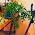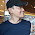### MATH 1280 Introduction to Statistics – Learning Journal 1

Textbook for Math 1280, credit to Amazon

It seems that everybody has no problem to download and install R program. We wish we could use the program during the study and in our everyday live.

2. Vocabulary and R functions
Enter the following command in R to read a simple help page about the table() command (this is for your information, you do not need to show the output):
?table
Now enter the following command and describe the output of the table() command. What does the first row of numbers in table() output represent? What does the second row of output represent?
x <- c(5, 8, 4, 1, 5, 6, 5, 9, 4, 2, 5, 7, 5, 3, 6, 4, 5, 3, 7, 6)
table(x)
[optional: You can test your theory by altering the numbers and rerunning the table command.]
x
1 2 3 4 5 6 7 8 9 ……> First row….> level of Input
1 1 2 3 6 3 2 1 1……> Second row….> frequency of each level input

The output of the function “table” is a table of the different levels of the input vector and the frequency of each level. This output is yet another type of an object. First row represents specifications such as discrete values.

Flowers, just for illustration

a) Read section 1.5 in the Yakir textbook. If you were a teacher and had 30 students in in your class and wanted to know the class average on the first quiz, would you use a parameter or a statistic? Why?
I will use parameter, since all 30 students in the class were included in the study (to calculate average grade of first quiz)

b) If you wanted to know how many people in your country recognize the name of you new company, would you use a parameter or a statistic? Why?
I will use statistic, it is because I will use part of country population to be surveyed.

1.Math and statistics. Two subjects that are well above my pay grade.

1.Thank you to visit my article:
MATH 1280 Introduction to Statistics – Learning Journal 1
@
Textbook for Math 1280, credit to Amazon

2.Thank you to visit my article:
MATH 1280 Introduction to Statistics – Learning Journal 1
@
Textbook for Math 1280, credit to Amazon

3.Thank you to visit my article:
MATH 1280 Introduction to Statistics – Learning Journal 1
@
Textbook for Math 1280, credit to Amazon

4.MATH 1280 Introduction to Statistics – Learning Journal 1
-
2. Vocabulary and R functions
Enter the following command in R to read a simple help page about the table() command (this is for your information, you do not need to show the output):
?table
Now enter the following command and describe the output of the table() command. What does the first row of numbers in table() output represent? What does the second row of output represent?
x <- c(5, 8, 4, 1, 5, 6, 5, 9, 4, 2, 5, 7, 5, 3, 6, 4, 5, 3, 7, 6)
table(x)
[optional: You can test your theory by altering the numbers and rerunning the table command.]
x
1 2 3 4 5 6 7 8 9 ……> First row….> level of Input
1 1 2 3 6 3 2 1 1……> Second row….> frequency of each level input

The output of the function “table” is a table of the different levels of the input vector and the frequency of each level. This output is yet another type of an object. First row represents specifications such as discrete values.

2.1.Thank you to visit my article:
MATH, 1280 Introduction to Statistics – Learning Journal 1
@
It seems that everybody has no problem to download and install R program. We wish we could use the program during the study and in our everyday live.

2.MATH, 1280 Introduction to Statistics – Learning Journal 1
@
It seems that everybody has no problem to download and install R program. We wish we could use the program during the study and in our everyday live.

3.MATH, 1280 Introduction to Statistics – Learning Journal 1
@
It seems that everybody has no problem to download and install R program. We wish we could use the program during the study and in our everyday live.

4.MATH 1280 Introduction to Statistics – Learning Journal 1
-
a) Read section 1.5 in the Yakir textbook. If you were a teacher and had 30 students in in your class and wanted to know the class average on the first quiz, would you use a parameter or a statistic? Why?
I will use parameter, since all 30 students in the class were included in the study (to calculate average grade of first quiz)

b) If you wanted to know how many people in your country recognize the name of you new company, would you use a parameter or a statistic? Why?
I will use statistic, it is because I will use part of country population to be surveyed.

3.It been a while since I been in class room.
Coffee is on

1.Thank you to visit my article:
MATH, 1280, Introduction to Statistics – Learning Journal 1
@
2. Vocabulary and R functions
Enter the following command in R to read a simple help page about the table() command (this is for your information, you do not need to show the output):
?table

2.MATH 1280 Introduction to Statistics – Learning Journal 1
@
2. Vocabulary and R functions
Enter the following command in R to read a simple help page about the table() command (this is for your information, you do not need to show the output):
?table
Now enter the following command and describe the output of the table() command. What does the first row of numbers in table() output represent? What does the second row of output represent?
x <- c(5, 8, 4, 1, 5, 6, 5, 9, 4, 2, 5, 7, 5, 3, 6, 4, 5, 3, 7, 6)
table(x)
[optional: You can test your theory by altering the numbers and rerunning the table command.]
x
1 2 3 4 5 6 7 8 9 ……> First row….> level of Input
1 1 2 3 6 3 2 1 1……> Second row….> frequency of each level input

The output of the function “table” is a table of the different levels of the input vector and the frequency of each level. This output is yet another type of an object. First row represents specifications such as discrete values.

3.MATH 1280 Introduction to Statistics – Learning Journal 1
@
a) Read section 1.5 in the Yakir textbook. If you were a teacher and had 30 students in in your class and wanted to know the class average on the first quiz, would you use a parameter or a statistic? Why?
I will use parameter, since all 30 students in the class were included in the study (to calculate average grade of first quiz)

b) If you wanted to know how many people in your country recognize the name of you new company, would you use a parameter or a statistic? Why?
I will use statistic, it is because I will use part of country population to be surveyed.

4.MATH 1280 Introduction to Statistics – Learning Journal 1
-
Textbook for Math 1280, credit to Amazon

4.Frankly, I'm not good at calculating lessons.
But there is nothing wrong with me being involved in reading and commenting on this post ... maybe I get smarter afterwards.

1.Thank you to visit my article:
MATH, 1280, Introduction, to Statistics – Learning Journal 1
@
Now enter the following command and describe the output of the table() command. What does the first row of numbers in table() output represent? What does the second row of output represent?
x <- c(5, 8, 4, 1, 5, 6, 5, 9, 4, 2, 5, 7, 5, 3, 6, 4, 5, 3, 7, 6)
table(x)
[optional: You can test your theory by altering the numbers and rerunning the table command.]
x
1 2 3 4 5 6 7 8 9 ……> First row….> level of Input
1 1 2 3 6 3 2 1 1……> Second row….> frequency of each level input

The output of the function “table” is a table of the different levels of the input vector and the frequency of each level. This output is yet another type of an object. First row represents specifications such as discrete values.

2.MATH, 1280, Introduction, to Statistics – Learning Journal 1
@
Now enter the following command and describe the output of the table() command. What does the first row of numbers in table() output represent? What does the second row of output represent?
x <- c(5, 8, 4, 1, 5, 6, 5, 9, 4, 2, 5, 7, 5, 3, 6, 4, 5, 3, 7, 6)
table(x)
[optional: You can test your theory by altering the numbers and rerunning the table command.]
x
1 2 3 4 5 6 7 8 9 ……> First row….> level of Input
1 1 2 3 6 3 2 1 1……> Second row….> frequency of each level input

The output of the function “table” is a table of the different levels of the input vector and the frequency of each level. This output is yet another type of an object. First row represents specifications such as discrete values.

3.MATH 1280 Introduction to Statistics – Learning Journal 1
@
Textbook for Math 1280, credit to Amazon

5.I had statistics in my university and I hated it. ;-)

Best regards

1.Thank you to visit my article:
MATH, 1280, Introduction, to, Statistics – Learning Journal 1
@
a) Read section 1.5 in the Yakir textbook. If you were a teacher and had 30 students in in your class and wanted to know the class average on the first quiz, would you use a parameter or a statistic? Why?
I will use parameter, since all 30 students in the class were included in the study (to calculate average grade of first quiz)

b) If you wanted to know how many people in your country recognize the name of you new company, would you use a parameter or a statistic? Why?
I will use statistic, it is because I will use part of country population to be surveyed.

2.MATH, 1280, Introduction, to, Statistics – Learning Journal 1
@
a) Read section 1.5 in the Yakir textbook. If you were a teacher and had 30 students in in your class and wanted to know the class average on the first quiz, would you use a parameter or a statistic? Why?
3.